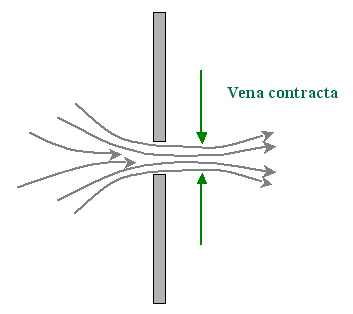•http://facebook.com/
•https://www.google.com/accounts/o8/id
•https://me.yahoo.com

# Introduction

Introduction to orifice

## Overview

An opening, in a vessel, through which the liquid flows out is known as orifice. This hole or opening is called an orifice, so long as the level of the liquid on the upstream side is above the top of the orifice.

The typical purpose of an orifice is the measurement of discharge. An orifice may be provided in the vertical side of a vessel or in the base. But the former one is more common.

## Types Of Orifices

Orifices can be of different types depending upon their size, shape, and nature of discharge. But the following are important from the subject point of view. A. According to size:
• Small orifice
• Large orifice
B. According to shape:
• Circular orifice
• Rectangular orifice
• Triangular orifice
C. According to shape of edge:
• Sharp-edged
• Bell-mouthed
D. According to nature of discharge:
• Fully submerged orifice
• Partially submerged orifice

## Jet Of Water

The continuous stream of a liquid, that comes out or flows out of an orifice, as known as the Jet of Water.

## Vena-contracta

Consider an orifice is fitted with a tank. The liquid particles, in order to flow out through the orifice, move towards the orifice from all directions. A few of the particles first move downward, then take a turn to enter into the orifice and then finally flow through it.Image Courtesy http://diracdelta.co.uk/science/source/v/e/vena%20contracta/source.html It may be noted, that the liquid particles loose some energy, while taking the turn to enter into the orifice. It can be observed that the jet, after leaving the orifice, gets contracted. The maximum contraction takes place at a section slightly on the downstream side of the orifice, where the jet is more or less horizontal. Such a section is known as Vena-Contracta.

## Hydraulic Coefficients

The following four coefficients are known as hydraulic coefficients or orifice coefficients.
• Coefficient of contraction
• Coefficient of velocity
• Coefficient of discharge
• Coefficient of resistance

## Coefficient Of Contraction

The ratio of the area of the jet, at vena-contracta, to the area of the orifice is known as coefficient of contraction. Mathematically coefficient of contraction,
The value of Coefficient of contraction varies slightly with the available head of the liquid, size and shape of the orifice. The average value of is 0.64.

## Coefficient Of Velocity

The ratio of actual velocity of the jet, at vena-contracta, to the theoretical velocity is known as coefficient of velocity.
The theoretical velocity of jet at vena-contracta is given by the relation, , where h is the head of water at vena-contracta.

Mathematically coefficient of velocity,

The difference between the velocities is due to friction of the orifice. The value of Coefficient of velocity varies slightly with the different shapes of the edges of the orifice. This value is very small for sharp-edged orifices. For a sharp edged orifice, the value of increases with the head of water.
##### Coefficient Of Discharge
The ratio of a actual discharge through an orifice to the theoretical discharge is known as coefficient of discharge. Mathematically coefficient of discharge,

Thus the value of coefficient of discharge varies with the values of and . An average of coefficient of discharge varies from 0.60 to 0.64.
##### Coefficient Of Resistance
The ratio of loss of head in the orifice to the head of water available at the exit of the orifice is known as coefficient of resistance.

The loss of head in the orifice takes place, because the walls of the orifice offer some resistance to the liquid as it comes out. The coefficient of resistance is generally neglected, while solving numerical problems.